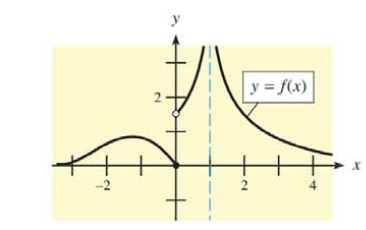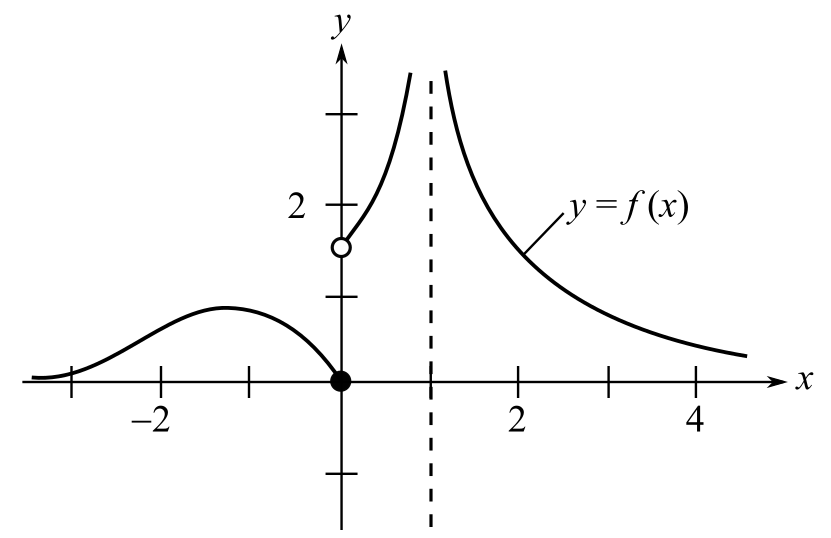Chapter 9, Problem 35REMathematical Applications for the ...

12th Edition
Ronald J. Harshbarger + 1 other
ISBN: 9781337625340

Solutions

Chapter
SectionMathematical Applications for the ...

12th Edition
Ronald J. Harshbarger + 1 other
ISBN: 9781337625340
Textbook Problem

In Problems 35 and 36, use the graphs to find (a) the points of discontinuity, (b) lim x → ∞ f ( x ) , and (c) lim x → − ∞ f ( x ) .(a)

To determine

The points of continuity for the function with the graph,Explanation

Given Information:

The provided graph of the function is

Explanation:

Consider the provided graph of the function,

For a function to be continuous at x=a the left hand limit must be equal to the right hand limit and the value of the function at x=a must be equal to the limits.

From the graph, the value of the function does not exist at x=0, as the point is indicated with a hollow circle

(b)

To determine

The value of limxf(x) from the graph of the function,(c)

To determine

The value of limxf(x) from the graph of the function,Still sussing out bartleby?

Check out a sample textbook solution.

See a sample solution

The Solution to Your Study Problems

Bartleby provides explanations to thousands of textbook problems written by our experts, many with advanced degrees!

Get Started

Find all possible real solutions of each equation in Exercises 3144. y3+64=0

Finite Mathematics and Applied Calculus (MindTap Course List)

Differentiate the function. f(x)=ln1x

Single Variable Calculus: Early Transcendentals, Volume I

In Exercises 99-106, factor out the greatest common factor from each expression. 99. 4x5 2x4 6x3

Applied Calculus for the Managerial, Life, and Social Sciences: A Brief Approach

Convert the following mixed numbers to improper fractions. 16.

Contemporary Mathematics for Business & Consumers

Evaluate the integral 39. 01/2xe2x(1+2x)2dx

Single Variable Calculus: Early Transcendentals

j × (−k) = i −i j + k −j − k

Study Guide for Stewart's Multivariable Calculus, 8th

The graph at the right is the direction field for: a) y = x y b) y = xy c) y = x + y d) y = xy

Study Guide for Stewart's Single Variable Calculus: Early Transcendentals, 8th